Learn data science and machine learning by building real-world projects on Jovian

DATA ANALYSIS : Automobile DatasetProblem

Let's say we have a friend named Tom. And Tom wants to sell his car. But the problem is he doesn't know how much he should sell his car for. Tom wants to sell his car for as much as he can. But he also wants to set the price reasonably, so someone would want to purchase it. So the price he sets should represent the value of the car.How can we help Tom determine the best price for his car? Let's think like data scientists and clearly define some of his problems. For example, is there data on the prices of other cars and their characteristics? What features of cars affect their prices? Color? Brand? Does horsepower also effect the selling price, or perhaps something else? As a data analyst or data scientist, these are some of the questions we can start thinking about. To answer these questions, we're going to need some data.

The final model has efficiency of 84% and below one is it's performance graph

DistributionPlot(y_test, yhat, "Actual Values (Test)", "Predicted Values (Test)", Title)
/opt/conda/lib/python3.8/site-packages/seaborn/distributions.py:2551: FutureWarning: `distplot` is a deprecated function and will be removed in a future version. Please adapt your code to use either `displot` (a figure-level function with similar flexibility) or `kdeplot` (an axes-level function for kernel density plots). warnings.warn(msg, FutureWarning) /opt/conda/lib/python3.8/site-packages/seaborn/distributions.py:2551: FutureWarning: `distplot` is a deprecated function and will be removed in a future version. Please adapt your code to use either `displot` (a figure-level function with similar flexibility) or `kdeplot` (an axes-level function for kernel density plots). warnings.warn(msg, FutureWarning)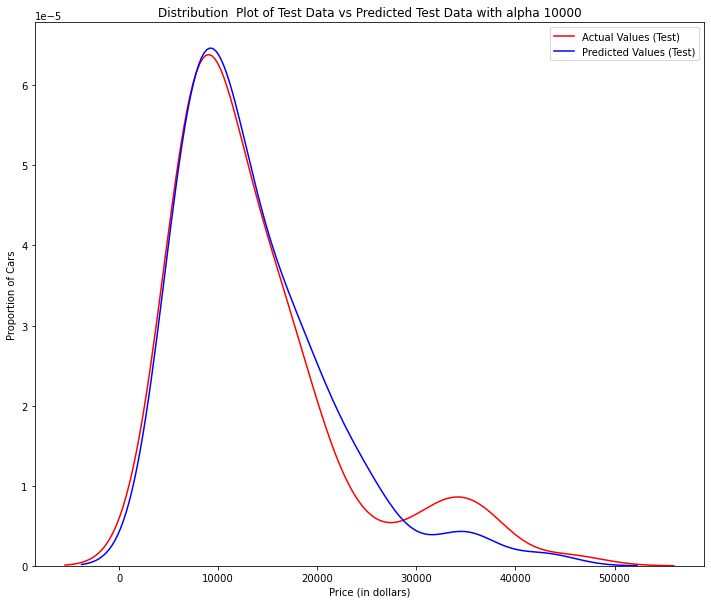TABLE OF CONTENT

1. Data Acquisition
2. Identify and handle missing values
3. Data Standardization
4. Data Normalization
5. Binning
6. Analyzing Individual Feature Patterns using Visualization
7. Model Development
• Linear Regression and Multiple Linear Regression
• Model Evaluation using Visualization
• Polynomial Regression and Pipeline
• Measures for Insample Evaluation
• Prediction and Decision Making
8. Model Evaluation and Refinement
9. Conclusion
10. Reference

1.Data Acquisition

There are various formats for a dataset, .csv, .json, .xlsx etc. The dataset can be stored in different places, on your local machine or sometimes online.In our case, the Automobile Dataset is an online source, and it is in CSV (comma separated value) format. Let's use this dataset as an example to practice data reading.

The Pandas Library is a useful tool that enables us to read various datasets into a data frame;so that all we need to do is import Pandas. If you crossed by error, install it first.

We use pandas.read_csv() function to read the csv file. In the bracket, we put the file path along with a quotation mark, so that pandas will read the file into a data frame from that address. The file path can be either an URL or your local file address.
Because the data does not include headers, we can add an argument headers = None inside the read_csv() method, so that pandas will not automatically set the first row as a header.
You can also assign the dataset to any variable you create.

! pip install ipywidgets --upgrade --quiet
import helper libraries
import pandas as pd
import numpy as np
import matplotlib.pyplot as plt
import seaborn as sns
from matplotlib import pyplot
%matplotlib inline
from scipy import stats
from sklearn.linear_model import LinearRegression
from sklearn.preprocessing import PolynomialFeatures
from sklearn.pipeline import Pipeline
from sklearn.preprocessing import StandardScaler
from sklearn.model_selection import train_test_split
from sklearn.metrics import r2_score
from sklearn.model_selection import cross_val_score
from sklearn.linear_model import Ridge
from sklearn.model_selection import GridSearchCV
from ipywidgets import interact, interactive, fixed, interact_manual
Read the online file by the URL provides above, and assign it to variable "df"
url = "https://cf-courses-data.s3.us.cloud-object-storage.appdomain.cloud/IBMDeveloperSkillsNetwork-DA0101EN-SkillsNetwork/labs/Data%20files/auto.csv"
After reading the dataset, we can use the dataframe.head(n) method to check the top n rows of the dataframe; where n is an integer.
# show the first 5 rows using dataframe.head() method
print("The first 5 rows of the dataframe")
The first 5 rows of the dataframe
Contrary to dataframe.head(n), dataframe.tail(n) will show you the bottom n rows of the dataframe.
print("The last 5 rows of the dataframe")
df.tail(10)
The last 5 rows of the dataframe

2.IDENTIFY AND HANDLE MISSING VALUES

Take a look at our dataset; pandas automatically set the header by an integer from 0.

To better describe our data we can introduce a header, this information is available at: https://archive.ics.uci.edu/ml/datasets/Automobile

Firstly, we create a list "headers" that include all column names in order. Then, we use dataframe.columns = headers to replace the headers by the list we created.

"drive-wheels","engine-location","wheel-base", "length","width","height","curb-weight","engine-type",
"num-of-cylinders", "engine-size","fuel-system","bore","stroke","compression-ratio","horsepower",
"peak-rpm","city-mpg","highway-mpg","price"]

View column names

df.columns
Index(['symboling', 'normalized-losses', 'make', 'fuel-type', 'aspiration',
'num-of-doors', 'body-style', 'drive-wheels', 'engine-location',
'wheel-base', 'length', 'width', 'height', 'curb-weight', 'engine-type',
'num-of-cylinders', 'engine-size', 'fuel-system', 'bore', 'stroke',
'compression-ratio', 'horsepower', 'peak-rpm', 'city-mpg',
'highway-mpg', 'price'],
dtype='object')

As we can see, several question marks appeared in the dataframe; those are missing values which may hinder our further analysis.

So, how do we identify all those missing values and deal with them?

How to work with missing data?

Steps for working with missing data:

1. identify missing data
2. deal with missing data
3. correct data format
we need to replace the "?" symbol with NaN so the dropna() can remove the missing values
df=df.replace('?',np.NaN)
df
Identify_missing_values
Evaluating for Missing Data

The missing values are converted to default. We use the following functions to identify these missing values. There are two methods to detect missing data:

1. .isnull()
2. .notnull()
The output is a boolean value indicating whether the value that is passed into the argument is in fact missing data.
missing_data = df.isnull()
True stands for missing value, while False stands for not missing value.
Count missing values in each column

Using a for loop in Python, we can quickly figure out the number of missing values in each column. As mentioned above, "True" represents a missing value, "False" means the value is present in the dataset. In the body of the for loop the method ".value_counts()" counts the number of "True" values.

for column in missing_data.columns:
print(column)
print (missing_data[column].value_counts())
print("")
symboling False 205 Name: symboling, dtype: int64 normalized-losses False 164 True 41 Name: normalized-losses, dtype: int64 make False 205 Name: make, dtype: int64 fuel-type False 205 Name: fuel-type, dtype: int64 aspiration False 205 Name: aspiration, dtype: int64 num-of-doors False 203 True 2 Name: num-of-doors, dtype: int64 body-style False 205 Name: body-style, dtype: int64 drive-wheels False 205 Name: drive-wheels, dtype: int64 engine-location False 205 Name: engine-location, dtype: int64 wheel-base False 205 Name: wheel-base, dtype: int64 length False 205 Name: length, dtype: int64 width False 205 Name: width, dtype: int64 height False 205 Name: height, dtype: int64 curb-weight False 205 Name: curb-weight, dtype: int64 engine-type False 205 Name: engine-type, dtype: int64 num-of-cylinders False 205 Name: num-of-cylinders, dtype: int64 engine-size False 205 Name: engine-size, dtype: int64 fuel-system False 205 Name: fuel-system, dtype: int64 bore False 201 True 4 Name: bore, dtype: int64 stroke False 201 True 4 Name: stroke, dtype: int64 compression-ratio False 205 Name: compression-ratio, dtype: int64 horsepower False 203 True 2 Name: horsepower, dtype: int64 peak-rpm False 203 True 2 Name: peak-rpm, dtype: int64 city-mpg False 205 Name: city-mpg, dtype: int64 highway-mpg False 205 Name: highway-mpg, dtype: int64 price False 201 True 4 Name: price, dtype: int64
Based on the summary above, each column has 205 rows of data, seven columns containing missing data:
1. "normalized-losses": 41 missing data
2. "num-of-doors": 2 missing data
3. "bore": 4 missing data
4. "stroke" : 4 missing data
5. "horsepower": 2 missing data
6. "peak-rpm": 2 missing data
7. "price": 4 missing data

Deal with missing data

How to deal with missing data?
1. drop data
a. drop the whole row
b. drop the whole column
2. replace data
a. replace it by mean
b. replace it by frequency
c. replace it based on other functions

3. Whole columns should be dropped only if most entries in the column are empty. In our dataset, none of the columns are empty enough to drop entirely. We have some freedom in choosing which method to replace data; however, some methods may seem more reasonable than others. We will apply each method to many different columns:

Replace by mean:

• "normalized-losses": 41 missing data, replace them with mean
• "stroke": 4 missing data, replace them with mean
• "bore": 4 missing data, replace them with mean
• "horsepower": 2 missing data, replace them with mean
• "peak-rpm": 2 missing data, replace them with mean

Replace by frequency:

• "num-of-doors": 2 missing data, replace them with "four".
• Reason: 84% sedans is four doors. Since four doors is most frequent, it is most likely to occur

Drop the whole row:

• "price": 4 missing data, simply delete the whole row
• Reason: price is what we want to predict. Any data entry without price data cannot be used for prediction; therefore any row now without price data is not useful to us
Replace by mean:
"normalized-losses": 41 missing data, replace them with mean
# Calculate the average of the column
avg_norm_loss = df["normalized-losses"].astype("float").mean(axis=0)
print("Average of normalized-losses:", avg_norm_loss)

#Replace "NaN" by mean value in "normalized-losses" column
df["normalized-losses"].replace(np.nan, avg_norm_loss, inplace=True)
Average of normalized-losses: 122.0
"bore": 4 missing data, replace them with mean
#Calculate the mean value for 'bore' column
avg_bore=df['bore'].astype('float').mean(axis=0)
print("Average of bore:", avg_bore)

#Replace NaN by mean value
df["bore"].replace(np.nan, avg_bore, inplace=True)
Average of bore: 3.3297512437810957
"stroke": 4 missing data, replace them with mean
#Calculate the mean vaule for "stroke" column
avg_stroke = df["stroke"].astype("float").mean(axis = 0)
print("Average of stroke:", avg_stroke)

#Replace "stroke" by mean value
df["stroke"].replace(np.nan, avg_stroke, inplace = True)
Average of stroke: 3.2554228855721337
"peak-rpm": 2 missing data, replace them with mean
#Calculate the mean vaule for "peak-rpm" column
avg_peak_rpm = df["peak-rpm"].astype("float").mean(axis = 0)
print("Average of peak-rpm:", avg_peak_rpm)

#Replace "peak-rpm" by mean value
df["peak-rpm"].replace(np.nan, avg_peak_rpm, inplace = True)
Average of peak-rpm: 5125.369458128079
"horsepower": 2 missing data, replace them with mean
#Calculate the mean vaule for "horsepower" column
avg_horsepower = df['horsepower'].astype('float').mean(axis=0)
print("Average horsepower:", avg_horsepower)

#Replace "horsepower" by mean value
df['horsepower'].replace(np.nan, avg_horsepower, inplace=True)
Average horsepower: 104.25615763546799
Replace by frequency:
"num-of-doors": 2 missing data

To see which values are present in a particular column, we can use the .value_counts() method:

df['num-of-doors'].value_counts()
four    114
two      89
Name: num-of-doors, dtype: int64

We can see that four doors are the most common type. We can also use the .idxmax() method to calculate for us the most common type automatically:

df['num-of-doors'].value_counts().idxmax()
'four'

The replacement procedure is very similar to what we have seen previously

#replace the missing 'num-of-doors' values by the most frequent
df["num-of-doors"].replace(np.nan, "four", inplace=True)
Finally, let's drop all rows that do not have price data:
# simply drop whole row with NaN in "price" column
df.dropna(subset=["price"], axis=0, inplace=True)

# reset index, because we droped two rows
df.reset_index(drop=True, inplace=True)

Correct data format

We are almost there!

The last step in data cleaning is checking and making sure that all data is in the correct format (int, float, text or other).

Data Types

Data has a variety of types.
The main types stored in Pandas dataframes are object, float, int, bool and datetime64. In order to better learn about each attribute, it is always good for us to know the data type of each column. In Pandas:

In Pandas, we use

.dtype() to check the data type

.astype() to change the data type

Lets list the data types for each column
df.dtypes
symboling              int64
normalized-losses     object
make                  object
fuel-type             object
aspiration            object
num-of-doors          object
body-style            object
drive-wheels          object
engine-location       object
wheel-base           float64
length               float64
width                float64
height               float64
curb-weight            int64
engine-type           object
num-of-cylinders      object
engine-size            int64
fuel-system           object
bore                  object
stroke                object
compression-ratio    float64
horsepower            object
peak-rpm              object
city-mpg               int64
highway-mpg            int64
price                 object
dtype: object

As we can see above, some columns are not of the correct data type. Numerical variables should have type 'float' or 'int', and variables with strings such as categories should have type 'object'. For example, 'bore' and 'stroke' variables are numerical values that describe the engines, so we should expect them to be of the type 'float' or 'int'; however, they are shown as type 'object'. We have to convert data types into a proper format for each column using the astype() method.

#Convert data types to proper format
df[["bore", "stroke","price","peak-rpm"]] = df[["bore", "stroke","price","peak-rpm"]].astype("float")
df[["normalized-losses"]] = df[["normalized-losses"]].astype("int")

Let us list the columns after the conversion

df.info(verbose = False)
<class 'pandas.core.frame.DataFrame'> RangeIndex: 201 entries, 0 to 200 Columns: 26 entries, symboling to price dtypes: float64(9), int64(6), object(11) memory usage: 41.0+ KB
df.info()
<class 'pandas.core.frame.DataFrame'> RangeIndex: 201 entries, 0 to 200 Data columns (total 26 columns): # Column Non-Null Count Dtype --- ------ -------------- ----- 0 symboling 201 non-null int64 1 normalized-losses 201 non-null int64 2 make 201 non-null object 3 fuel-type 201 non-null object 4 aspiration 201 non-null object 5 num-of-doors 201 non-null object 6 body-style 201 non-null object 7 drive-wheels 201 non-null object 8 engine-location 201 non-null object 9 wheel-base 201 non-null float64 10 length 201 non-null float64 11 width 201 non-null float64 12 height 201 non-null float64 13 curb-weight 201 non-null int64 14 engine-type 201 non-null object 15 num-of-cylinders 201 non-null object 16 engine-size 201 non-null int64 17 fuel-system 201 non-null object 18 bore 201 non-null float64 19 stroke 201 non-null float64 20 compression-ratio 201 non-null float64 21 horsepower 201 non-null object 22 peak-rpm 201 non-null float64 23 city-mpg 201 non-null int64 24 highway-mpg 201 non-null int64 25 price 201 non-null float64 dtypes: float64(9), int64(6), object(11) memory usage: 41.0+ KB
Wonderful!

Now, we finally obtain the cleaned dataset with no missing values and all data in its proper format.

Data Standardization

Data is usually collected from different agencies with different formats. (Data Standardization is also a term for a particular type of data normalization, where we subtract the mean and divide by the standard deviation)

What is Standardization?

Standardization is the process of transforming data into a common format which allows the researcher to make the meaningful comparison.

Example

Transform mpg to L/100km:

In our dataset, the fuel consumption columns "city-mpg" and "highway-mpg" are represented by mpg (miles per gallon) unit. Assume we are developing an application in a country that accept the fuel consumption with L/100km standard

We will need to apply data transformation to transform mpg into L/100km?

The formula for unit conversion is

L/100km = 235 / mpg

We can do many mathematical operations directly in Pandas.

# Convert mpg to L/100km by mathematical operation (235 divided by mpg)
df['city-L/100km'] = 235/df["city-mpg"]
df["highway-L/100km"] = 235/df["highway-mpg"]

Data Normalization

Why normalization?

Normalization is the process of transforming values of several variables into a similar range. Typical normalizations include scaling the variable so the variable average is 0, scaling the variable so the variance is 1, or scaling variable so the variable values range from 0 to 1

Example

To demonstrate normalization, let's say we want to scale the columns "length", "width" and "height"

Target:would like to Normalize those variables so their value ranges from 0 to 1.

Approach: replace original value by (original value)/(maximum value)

df[["length","width","height"]].head() # these values vary highly w.r.t rest column values
# replace (original value) by (original value)/(maximum value) -> Simple Feature Scaling
df['length'] = df['length']/df['length'].max()
df['width'] = df['width']/df['width'].max()
df['height'] = df['height']/df['height'].max()

# show the scaled columns

Binning

Why binning?

Binning is a process of transforming continuous numerical variables into discrete categorical 'bins', for grouped analysis.

Example:

In our dataset, "horsepower" is a real valued variable ranging from 48 to 288, it has 57 unique values. What if we only care about the price difference between cars with high horsepower, medium horsepower, and little horsepower (3 types)? Can we rearrange them into three ‘bins' to simplify analysis?

We will use the Pandas method 'cut' to segment the 'horsepower' column into 3 bins

Example of Binning Data In Pandas

Convert data to correct format

Lets plot the histogram of horspower, to see what the distribution of horsepower looks like.

df["horsepower"]=df["horsepower"].astype(int, copy=True)
plt.hist(df["horsepower"])

# set x/y labels and plot title
plt.xlabel("horsepower")
plt.ylabel("count")
plt.title("horsepower bins")
Text(0.5, 1.0, 'horsepower bins')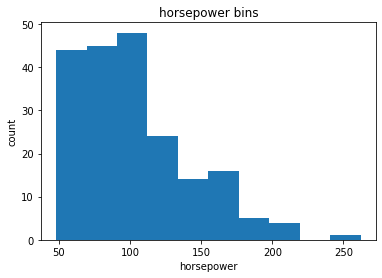We would like 3 bins of equal size bandwidth so we use numpy's linspace(start_value, end_value, numbers_generated) function.

Since we want to include the minimum value of horsepower we want to set start_value=min(df["horsepower"]).

Since we want to include the maximum value of horsepower we want to set end_value=max(df["horsepower"]).

Since we are building 3 bins of equal length, there should be 4 dividers, so numbers_generated=4.

We build a bin array, with a minimum value to a maximum value, with bandwidth calculated above. The bins will be values used to determine when one bin ends and another begins.

bins = np.linspace(min(df["horsepower"]), max(df["horsepower"]), 4)
bins
array([ 48.        , 119.33333333, 190.66666667, 262.        ])

We set group names:

group_names = ['Low', 'Medium', 'High']

We apply the function cut that determine what each value of df['horsepower'] belongs to.

df['horsepower-binned'] = pd.cut(df['horsepower'], bins, labels=group_names, include_lowest=True )

#Lets see the number of vehicles in each bin
df["horsepower-binned"].value_counts()
Low       153
Medium     43
High        5
Name: horsepower-binned, dtype: int64

Lets plot the distribution of each bin.

pyplot.bar(group_names, df["horsepower-binned"].value_counts())

# set x/y labels and plot title
plt.xlabel("horsepower")
plt.ylabel("count")
plt.title("horsepower bins")
Text(0.5, 1.0, 'horsepower bins')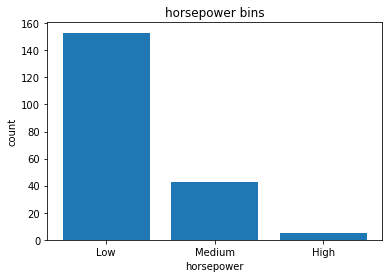Check the dataframe above carefully, you will find the last column provides the bins for "horsepower" with 3 categories ("Low","Medium" and "High")

Bins visualization

Normally, a histogram is used to visualize the distribution of bins we created above.
# draw historgram of attribute "horsepower" with bins = 3
plt.hist(df["horsepower"], bins = 3)

# set x/y labels and plot title
plt.xlabel("horsepower")
plt.ylabel("count")
plt.title("horsepower bins")
Text(0.5, 1.0, 'horsepower bins')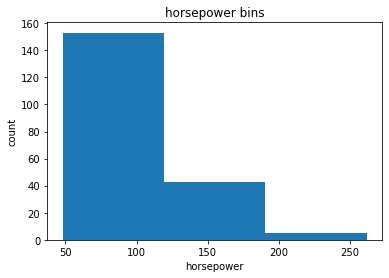Indicator variable (or dummy variable)

What is an indicator variable?

An indicator variable (or dummy variable) is a numerical variable used to label categories. They are called 'dummies' because the numbers themselves don't have inherent meaning.

Why we use indicator variables?

So we can use categorical variables for regression analysis in the later modules.

Example

We see the column "fuel-type" has two unique values, "gas" or "diesel". Regression doesn't understand words, only numbers. To use this attribute in regression analysis, we convert "fuel-type" into indicator variables.

We will use the panda's method 'get_dummies' to assign numerical values to different categories of fuel type.

get indicator variables as fuel-type and assign it to data frame dummy_variable_1

dummy_variable_1 = pd.get_dummies(df["fuel-type"])
dummy_variable_1.sample(5)

change column names for clarity

dummy_variable_1.rename(columns={'gas':'fuel-type-gas', 'diesel':'fuel-type-diesel'}, inplace=True)

In the dataframe, column fuel-type has a value for 'gas' and 'diesel'as 0s and 1s now

# merge data frame "df" and "dummy_variable_1"
df = pd.concat([df, dummy_variable_1], axis=1)

# drop original column "fuel-type" from "df"
df.drop("fuel-type", axis = 1, inplace=True)

Repeat for aspiration column

# get indicator variables of aspiration and assign it to data frame "dummy_variable_2"
dummy_variable_2 = pd.get_dummies(df['aspiration'])

# change column names for clarity
dummy_variable_2.rename(columns={'std':'aspiration-std', 'turbo': 'aspiration-turbo'}, inplace=True)

# show first 5 instances of data frame "dummy_variable_1"
# merge data frame "df" and "dummy_variable_1"
df = pd.concat([df, dummy_variable_2], axis=1)

# drop original column "fuel-type" from "df"
df.drop("aspiration", axis = 1, inplace=True)

Analyzing Individual Feature Patterns using Visualization

How to choose the right visualization method?
When visualizing individual variables, it is important to first understand what type of variable you are dealing with. This will help us find the right visualization method for that variable.
# list the data types for each column
print(df.dtypes)
symboling int64 normalized-losses int64 make object num-of-doors object body-style object drive-wheels object engine-location object wheel-base float64 length float64 width float64 height float64 curb-weight int64 engine-type object num-of-cylinders object engine-size int64 fuel-system object bore float64 stroke float64 compression-ratio float64 horsepower int64 peak-rpm float64 city-mpg int64 highway-mpg int64 price float64 city-L/100km float64 highway-L/100km float64 horsepower-binned category fuel-type-diesel uint8 fuel-type-gas uint8 aspiration-std uint8 aspiration-turbo uint8 dtype: object

1. Continuous numerical variables:

Continuous numerical variables are variables that may contain any value within some range. Continuous numerical variables can have the type int64 or float64. A great way to visualize these variables is by using scatterplots with fitted lines.

In order to start understanding the (linear) relationship between an individual variable and the price. We can do this by using "regplot", which plots the scatterplot plus the fitted regression line for the data.

Positive linear relationship
Let's find the scatterplot of "engine-size" and "price"
sns.regplot(x = 'engine-size', y = 'price', data = df)
plt.ylim(0,) # y axis starts from zero
plt.title("correlation between engine-size and price")
plt.show()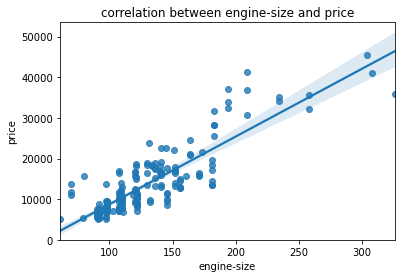As the engine-size goes up, the price goes up: this indicates a positive direct correlation between these two variables. Engine size seems like a pretty good predictor of price since the regression line is almost a perfect diagonal line.We can examine the correlation between engine-size and price and see it's approximately 0.87

we can calculate the correlation between variables of type int64 or float64 using the method corr: The diagonal elements are always one
df[["engine-size", "price"]].corr()
Highway mpg is a potential predictor variable of price

Highway MPG: the average a car will get while driving on an open stretch of road without stopping or starting, typically at a higher speed. City MPG: the score a car will get on average in city conditions, with stopping and starting at lower speeds.

sns.regplot(x = 'highway-mpg', y = 'price',data = df)
print('correlation between highway-mpg and price ')
df[['highway-mpg', 'price']].corr()
correlation between highway-mpg and price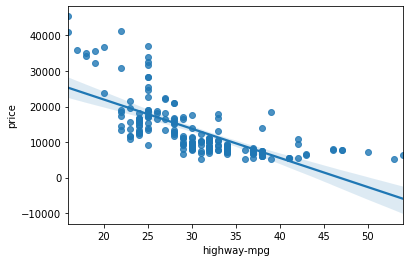As the highway-mpg goes up, the price goes down: this indicates an inverse/negative relationship between these two variables. Highway mpg could potentially be a predictor of price.We can examine the correlation between highway-mpg and price and see it's approximately -0.704

Weak Linear Relationship
Let's see if Peak-rpm as a predictor variable of price
sns.regplot(x = 'peak-rpm', y = 'price', data = df)
print('correlation between peak-rpm and price ')
df[['peak-rpm','price']].corr()
correlation between peak-rpm and price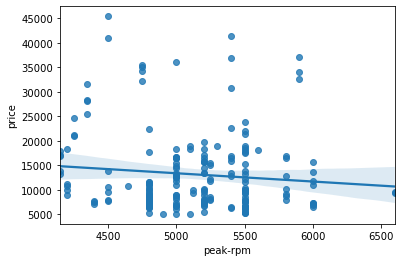Peak rpm does not seem like a good predictor of the price at all since the regression line is close to horizontal. Also, the data points are very scattered and far from the fitted line, showing lots of variability. Therefore it's it is not a reliable variable.We can examine the correlation between peak-rp and price and see it's approximately -0.101616

Let's see if stroke as a predictor variable of price
print('correlation between strike and prce :')

# correlation results between "price" and "stroke" do you expect a linear relationship?
sns.regplot(x = 'stroke', y = 'price', data = df)

# correlation between x="stroke", y="price".
df[['stroke','price']].corr()
correlation between strike and prce :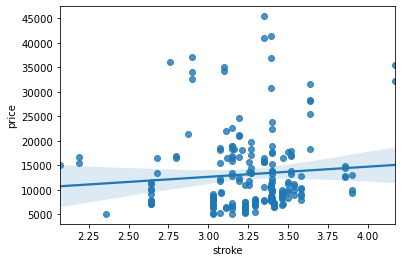Stroke does not seem like a good predictor of the price at all since the regression line is close to horizontal. Also, the data points are very scattered and far from the fitted line, showing lots of variability. Therefore it's it is not a reliable variable.We can examine the correlation between stroke and price and see it's approximately 0.08

2. Categorical variables

These are variables that describe a characteristic of a data unit, and are selected from a small group of categories. The categorical variables can have the type object or int64. A good way to visualize categorical variables is by using boxplots.

Let's look at the relationship between body-style and price.
sns.boxplot(x="body-style", y="price", data=df)
<AxesSubplot:xlabel='body-style', ylabel='price'>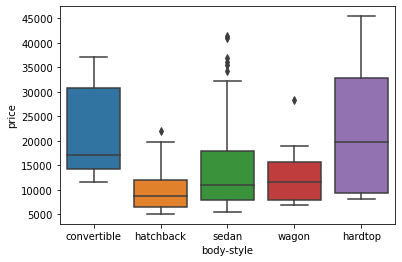We see that the distributions of price between the different body-style categories have a significant overlap, and so body-style would not be a good predictor of price.

Let's examine if engine-location as a predictor variable of price :
sns.boxplot(x = 'engine-location', y = 'price', data = df)
<AxesSubplot:xlabel='engine-location', ylabel='price'>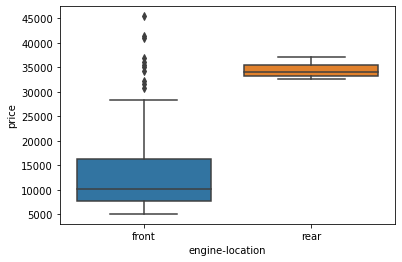Here we see that the distribution of price between these two engine-location categories, front and rear, are distinct enough to take engine-location as a potential good predictor of price.

Let's examine if drive-wheels as a predictor variable of price :

A drive wheel is a wheel of a motor vehicle that transmits force, transforming torque into tractive force from the tires to the road, causing the vehicle to move.

sns.boxplot(x = 'drive-wheels', y = 'price', data = df)
<AxesSubplot:xlabel='drive-wheels', ylabel='price'>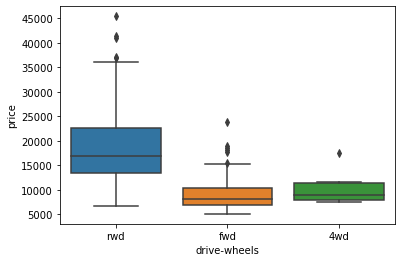Here we see that the distribution of price between the different drive-wheels categories differs; as such drive-wheels could potentially be a predictor of price.

3. Descriptive Statistical Analysis

Let's first take a look at the variables by utilizing a description method.

The describe function automatically computes basic statistics for all continuous variables. Any NaN values are automatically skipped in these statistics.

This will show:

• the count of that variable
• the mean
• the standard deviation (std)
• the minimum value
• the IQR (Interquartile Range: 25%, 50% and 75%)
• the maximum value
We can apply the method describe as follows:
df.describe()
The default setting of describe skips variables of type object. We can apply the method describe on the variables of type object as follows:
df.describe(include = ['object'])
We can apply the method describe on the variables of type all datatypes as follows:
df.describe(include = 'all')

Value Counts

Value-counts is a good way of understanding how many units of each characteristic/variable we have. We can apply the value_counts method on the column 'drive-wheels'. Don’t forget the method value_counts only works on Pandas series, not Pandas Dataframes. As a result, we only include one bracket df['drive-wheels'] not two brackets df[['drive-wheels']].
df['drive-wheels'].value_counts()
fwd    118
rwd     75
4wd      8
Name: drive-wheels, dtype: int64

We can convert the series to a Dataframe as follows :

df['drive-wheels'].value_counts().to_frame()

Let's repeat the above steps but save the results to the dataframe drive_wheels_counts and rename the column drive-wheels to value_counts.

A vehicle's drive wheel is the wheel and tire assembly that actually pushes or pulls the vehicle down the road.The four different types of drivetrain are all-wheel-drive (AWD), front wheel drive (FWD), rear wheel drive (RWD), and 4WD (4 wheel drive). (more)

drive_wheels_counts = df['drive-wheels'].value_counts().to_frame()
drive_wheels_counts.rename(columns={'drive-wheels': 'value_counts'}, inplace=True)
drive_wheels_counts.index.name = 'drive-wheels' # Now let's rename the index to 'drive-wheels':
drive_wheels_counts

We can repeat the above process for the variable engine-location.

engine_location_counts = df['engine-location'].value_counts().to_frame()
engine_location_counts.rename(columns = {'engine-location' : 'value_counts'}, inplace = True)
engine_location_counts.index.name = 'engine-location'
engine_location_counts

Examining the value counts of the engine location would not be a good predictor variable for the price. This is because we only have three cars with a rear engine and 198 with an engine in the front, this result is skewed. Thus, we are not able to draw any conclusions about the engine location.

4.Basics of Grouping

The groupby method groups data by different categories. The data is grouped based on one or several variables and analysis is performed on the individual groups.

For example, let's group by the variable drive-wheels. We see that there are 3 different categories of drive wheels.

For example, let's group by the variable drive-wheels. We see that there are 3 different categories of drive wheels.

df["drive-wheels"].unique()
array(['rwd', 'fwd', '4wd'], dtype=object)

If we want to know, on average, which type of drive wheel is most valuable, we can group drive-wheels and then average them.

We can select the columns drive-wheels, body-style and price, then assign it to the variable df_group_one.

We can then calculate the average price for each of the different categories of data.
df_group_one = df[['drive-wheels', 'body-style', 'price']]
df_group_one = df_group_one.groupby(['drive-wheels'],as_index = False).mean() # if not passes as_index False it will make drive wheels as index
df_group_one

From our data, it seems rear-wheel drive vehicles are, on average, the most expensive, while 4-wheel and front-wheel are approximately the same in price.

You can also group with multiple variables. For example, let's group by both drive-wheels and body-style. This groups the dataframe by the unique combinations drive-wheels and body-style. We can store the results in the variable 'grouped_test1'.

# grouping results
df_gptest = df[['drive-wheels','body-style','price']]
grouped_test1 = df_gptest.groupby(['drive-wheels','body-style'],as_index=False).mean()
grouped_test1This grouped data is much easier to visualize when it is made into a pivot table. A pivot table is like an Excel spreadsheet, with one variable along the column and another along the row. We can convert the dataframe to a pivot table using the method "pivot " to create a pivot table from the groups.

In this case, we will leave the drive-wheel variable as the rows of the table, and pivot body-style to become the columns of the table:

grouped_pivot = grouped_test1.pivot(index='drive-wheels',columns='body-style')
grouped_pivot

Often, we won't have data for some of the pivot cells. We can fill these missing cells with the value 0, but any other value could potentially be used as well. It should be mentioned that missing data is quite a complex subject and is an entire course on its own. For simplicity, let's assign them 0

grouped_pivot = grouped_pivot.fillna(0) #fill missing values with 0
grouped_pivot
Use the groupby function to find the average price of each car based on body-style ?
df_gptest2 = df[['body-style','price']]
grouped_test_bodystyle = df_gptest2.groupby(['body-style'],as_index= False).mean()
grouped_test_bodystyle
Variables: Drive Wheels and Body Style vs Price
Let's use a heat map to visualize the relationship between Body Style vs Price.
plt.pcolor(grouped_pivot, cmap = 'RdBu')
plt.colorbar() # show vertical range
plt.show()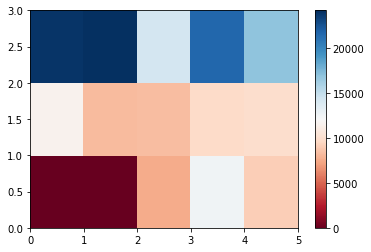The heatmap plots the target variable (price) proportional to colour with respect to the variables 'drive-wheel' and 'body-style' in the vertical and horizontal axis respectively. This allows us to visualize how the price is related to 'drive-wheel' and 'body-style'.

The default labels convey no useful information to us. Let's change that:

grouped_pivot.index
Index(['4wd', 'fwd', 'rwd'], dtype='object', name='drive-wheels')
fig, ax = plt.subplots()
im = ax.pcolor(grouped_pivot, cmap='RdBu')

#label names
row_labels = grouped_pivot.columns.levels # accesing "body-type" from grouped.pivot
col_labels = grouped_pivot.index # # accesing "drive-wheels" from grouped.pivot

#move ticks and labels to the center
ax.set_xticks(np.arange(grouped_pivot.shape) + 0.5, minor=False)
ax.set_yticks(np.arange(grouped_pivot.shape) + 0.5, minor=False)

#insert labels
ax.set_xticklabels(row_labels, minor=False)
ax.set_yticklabels(col_labels, minor=False)

#rotate label if too long
plt.xticks(rotation=90)

fig.colorbar(im)
plt.show()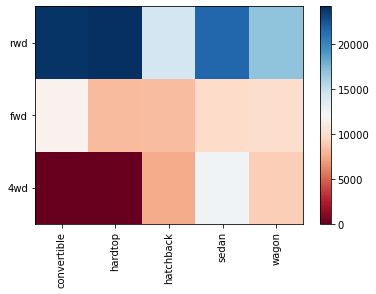5. Correlation and Causation

Correlation: a measure of the extent of interdependence between variables.

Causation: the relationship between cause and effect between two variables.

It is important to know the difference between these two and that correlation does not imply causation. Determining correlation is much simpler the determining causation as causation may require independent experimentation.

Pearson Correlation

The Pearson Correlation measures the linear dependence between two variables X and Y.

The resulting coefficient is a value between -1 and 1 inclusive, where:

• 1: Total positive linear correlation.
• 0: No linear correlation, the two variables most likely do not affect each other.
• -1: Total negative linear correlation.
df.corr()
sometimes we would like to know the significant of the correlation estimate.
P-value:

What is this P-value? The P-value is the probability value that the correlation between these two variables is statistically significant. Normally, we choose a significance level of 0.05, which means that we are 95% confident that the correlation between the variables is significant.

By convention, when the

• p-value is \$<\$ 0.001: we say there is strong evidence that the correlation is significant.
• the p-value is \$<\$ 0.05: there is moderate evidence that the correlation is significant.
• the p-value is \$<\$ 0.1: there is weak evidence that the correlation is significant.
• the p-value is \$>\$ 0.1: there is no evidence that the correlation is significant.

We can obtain this information using stats module in the scipy library.

Wheel-base vs Price

Let's calculate the Pearson Correlation Coefficient and P-value of wheel-base and price.

pearson_coef, p_value = stats.pearsonr(df['wheel-base'], df['price'])
print("The Pearson Correlation Coefficient is", pearson_coef, " with a P-value of P =", p_value)
The Pearson Correlation Coefficient is 0.5846418222655085 with a P-value of P = 8.076488270732243e-20

Conclusion:

Since the p-value is < 0.001, the correlation between wheel-base and price is statistically significant, although the linear relationship isn't extremely strong (~0.585)

Horsepower vs Price

Let's calculate the Pearson Correlation Coefficient and P-value of horsepower and price.

pearson_coef, p_value = stats.pearsonr(df['horsepower'], df['price'])
print("The Pearson Correlation Coefficient is", pearson_coef, " with a P-value of P = ", p_value)
The Pearson Correlation Coefficient is 0.8096068016571051 with a P-value of P = 6.273536270651218e-48

Conclusion:

Since the p-value is < 0.001, the correlation between horsepower and price is statistically significant, and the linear relationship is quite strong (~0.809, close to 1)

Length vs Price

Let's calculate the Pearson Correlation Coefficient and P-value of length and price.

pearson_coef, p_value = stats.pearsonr(df['length'], df['price'])
print("The Pearson Correlation Coefficient is", pearson_coef, " with a P-value of P = ", p_value)
The Pearson Correlation Coefficient is 0.6906283804483644 with a P-value of P = 8.016477466158188e-30

Conclusion:

Since the p-value is < 0.001, the correlation between length and price is statistically significant, and the linear relationship is moderately strong (~0.691).

Width vs Price

Let's calculate the Pearson Correlation Coefficient and P-value of width and price.

pearson_coef, p_value = stats.pearsonr(df['width'], df['price'])
print("The Pearson Correlation Coefficient is", pearson_coef, " with a P-value of P =", p_value )
The Pearson Correlation Coefficient is 0.7512653440522674 with a P-value of P = 9.200335510481516e-38

Conclusion:

Since the p-value is < 0.001, the correlation between width and price is statistically significant, and the linear relationship is quite strong (~0.751).

Curb-weight vs Price

Let's calculate the Pearson Correlation Coefficient and P-value of curb-weight and price.

pearson_coef, p_value = stats.pearsonr(df['curb-weight'], df['price'])
print( "The Pearson Correlation Coefficient is", pearson_coef, " with a P-value of P = ", p_value)
The Pearson Correlation Coefficient is 0.8344145257702845 with a P-value of P = 2.189577238893816e-53

Conclusion:

Since the p-value is < 0.001, the correlation between curb-weight and price is statistically significant, and the linear relationship is quite strong (~0.834)

Engine-size vs Price

Let's calculate the Pearson Correlation Coefficient and P-value of engine-size and price.

pearson_coef, p_value = stats.pearsonr(df['engine-size'], df['price'])
print("The Pearson Correlation Coefficient is", pearson_coef, " with a P-value of P =", p_value)
The Pearson Correlation Coefficient is 0.8723351674455188 with a P-value of P = 9.265491622196808e-64

Conclusion:

Since the p-value is < 0.001, the correlation between engine-size and price is statistically significant, and the linear relationship is very strong (~0.872).

Bore vs Price

Let's calculate the Pearson Correlation Coefficient and P-value of bore and price.

pearson_coef, p_value = stats.pearsonr(df['bore'], df['price'])
print("The Pearson Correlation Coefficient is", pearson_coef, " with a P-value of P =  ", p_value )
The Pearson Correlation Coefficient is 0.54315538326266 with a P-value of P = 8.049189483935489e-17

Conclusion:

Since the p-value is < 0.001, the correlation between bore and price is statistically significant, but the linear relationship is only moderate (~0.521).

City-mpg vs Price

Let's calculate the Pearson Correlation Coefficient and P-value of city-mpg and price.

pearson_coef, p_value = stats.pearsonr(df['city-mpg'], df['price'])
print("The Pearson Correlation Coefficient is", pearson_coef, " with a P-value of P = ", p_value)
The Pearson Correlation Coefficient is -0.6865710067844684 with a P-value of P = 2.3211320655672453e-29

Conclusion:

Since the p-value is < 0.001, the correlation between city-mpg and price is statistically significant, and the coefficient of ~ -0.687 shows that the relationship is negative and moderately strong.

Highway-mpg vs Price¶

Let's calculate the Pearson Correlation Coefficient and P-value of highway-mpg and price.

pearson_coef, p_value = stats.pearsonr(df['highway-mpg'], df['price'])
print( "The Pearson Correlation Coefficient is", pearson_coef, " with a P-value of P = ", p_value )
The Pearson Correlation Coefficient is -0.7046922650589534 with a P-value of P = 1.749547114447437e-31

Conclusion:

Since the p-value is < 0.001, the correlation between highway-mpg and price is statistically significant, and the coefficient of ~ -0.705 shows that the relationship is negative and moderately strong.

6. ANOVA

The Analysis of Variance (ANOVA) is a statistical method used to test whether there are significant differences between the means of two or more groups. ANOVA returns two parameters:

F-test score: ANOVA assumes the means of all groups are the same, calculates how much the actual means deviate from the assumption, and reports it as the F-test score. A larger score means there is a larger difference between the means.

P-value: P-value tells how statistically significant is our calculated score value.

If our price variable is strongly correlated with the variable we are analyzing, expect ANOVA to return a sizeable F-test score and a small p-value.

Drive Wheels

Since ANOVA analyzes the difference between different groups of the same variable, the groupby function will come in handy. Because the ANOVA algorithm averages the data automatically, we do not need to take the average before hand.

Let's see if different types drive-wheels impact price, we group the data.

grouped_test2=df_gptest[['drive-wheels', 'price']].groupby(['drive-wheels'])

We can obtain the values of the method group using the method get_group.

grouped_test2.get_group('4wd')['price']
4      17450.0
136     7603.0
140     9233.0
141    11259.0
144     8013.0
145    11694.0
150     7898.0
151     8778.0
Name: price, dtype: float64

we can use the function f_oneway in the module stats to obtain the F-test score and P-value.

# ANOVA
f_val, p_val = stats.f_oneway(grouped_test2.get_group('fwd')['price'], grouped_test2.get_group('rwd')['price'], grouped_test2.get_group('4wd')['price'])

print( "ANOVA results: F=", f_val, ", P =", p_val)
ANOVA results: F= 67.95406500780399 , P = 3.3945443577151245e-23

This is a great result, with a large F test score showing a strong correlation and a P value of almost 0 implying almost certain statistical significance. But does this mean all three tested groups are all this highly correlated?

Separately:

f_val, p_val = stats.f_oneway(grouped_test2.get_group('fwd')['price'], grouped_test2.get_group('rwd')['price'])
print( "fwd and rwd -> ANOVA results: F=", f_val, ", P =", p_val )

f_val, p_val = stats.f_oneway(grouped_test2.get_group('4wd')['price'], grouped_test2.get_group('rwd')['price'])
print( "4wd and rwd -> ANOVA results: F=", f_val, ", P =", p_val)

f_val, p_val = stats.f_oneway(grouped_test2.get_group('4wd')['price'], grouped_test2.get_group('fwd')['price'])
print("4wd and fwd -> ANOVA results: F=", f_val, ", P =", p_val)
fwd and rwd -> ANOVA results: F= 130.5533160959111 , P = 2.2355306355677845e-23 4wd and rwd -> ANOVA results: F= 8.580681368924756 , P = 0.004411492211225333 4wd and fwd -> ANOVA results: F= 0.665465750252303 , P = 0.41620116697845666

Conclusion: Important Variables

We now have a better idea of what our data looks like and which variables are important to take into account when predicting the car price. We have narrowed it down to the following variables:

Continuous numerical variables:

• Length
• Width
• Curb-weight
• Engine-size
• Horsepower
• City-mpg
• Highway-mpg
• Wheel-base
• Bore

Categorical variables:

• Drive-wheels

As we now move into building machine learning models to automate our analysis, feeding the model with variables that meaningfully affect our target variable will improve our model's prediction performance.

7. Model Development

Objectives

Develop prediction models

In this section, we will develop several models that will predict the price of the car using the variables or features. This is just an estimate but should give us an objective idea of how much the car should cost.

Some questions we want to ask in this module

• do I know if the dealer is offering fair value for my trade-in?
• do I know if I put a fair value on my car?
• Data Analytics, we often use Model Development to help us predict future observations from the data we have.

A Model will help us understand the exact relationship between different variables and how these variables are used to predict the result.

1: Linear Regression and Multiple Linear Regression

Linear Regression

One example of a Data Model that we will be using is

Simple Linear Regression.

Simple Linear Regression is a method to help us understand the relationship between two variables:

• The predictor/independent variable (X)
• The response/dependent variable (that we want to predict)(Y)

The result of Linear Regression is a linear function that predicts the response (dependent) variable as a function of the predictor (independent) variable.

\[ Y: Response \ Variable\\ X: Predictor \ Variables \]

Linear function: \[ Yhat = a + b X \]

• a refers to the intercept of the regression line0, in other words: the value of Y when X is 0
• b refers to the slope of the regression line, in other words: the value with which Y changes when X increases by 1 unit

Create the linear regression object

lm = LinearRegression()

How could Highway-mpg help us predict car price?

we will create a linear function with "highway-mpg" as the predictor variable and the "price" as the response variable.

X = df[['highway-mpg']]
Y = df['price']

Fit the linear model using highway-mpg.

lm.fit(X,Y)
LinearRegression()

We can output a prediction

Yhat=lm.predict(X)
Yhat[0:5]
array([16236.50464347, 16236.50464347, 17058.23802179, 13771.3045085 ,
20345.17153508])

What is the value of the intercept (a)?

lm.intercept_
38423.30585815743

What is the value of the Slope (b)?

lm.coef_
array([-821.73337832])

What is the final estimated linear model we get?

As we saw above, we should get a final linear model with the structure:

\[ Yhat = a + b X \]

with actual values we get: price = 38423.31 - 821.73 * highway-mpg

Train the model using 'engine-size' as the independent variable and 'price' as the dependent variable

# Extracting independent variable target variables
lm1 = LinearRegression()

# fit in linear model
lm1.fit( df[['engine-size']], df['price'])

print("What is the value of the intercept (a)? \n {}".format(lm1.intercept_))
print("What is the value of the Slope (b)? \n {}".format(lm1.coef_))
print("\n Final estimated linear model")
print("Yhat=-7963.34 + 166.86*X")
print("Price=-7963.34 + 166.86*engine-size")
What is the value of the intercept (a)? -7963.338906281049 What is the value of the Slope (b)? [166.86001569] Final estimated linear model Yhat=-7963.34 + 166.86*X Price=-7963.34 + 166.86*engine-size

Multiple Linear Regression

What if we want to predict car price using more than one variable?

If we want to use more variables in our model to predict car price, we can use Multiple Linear Regression. Multiple Linear Regression is very similar to Simple Linear Regression, but this method is used to explain the relationship between one continuous response (dependent) variable and two or more predictor (independent) variables. Most of the real-world regression models involve multiple predictors. We will illustrate the structure by using four predictor variables, but these results can generalize to any integer:

\[ Y: Response \ Variable\\ X_1 :Predictor \ Variable \ 1\\ X_2: Predictor\ Variable \ 2\\ X_3: Predictor\ Variable \ 3\\ X_4: Predictor\ Variable \ 4\\ \]

\[ a: intercept\\ b_1 :coefficients \ of\ Variable \ 1\\ b_2: coefficients \ of\ Variable \ 2\\ b_3: coefficients \ of\ Variable \ 3\\ b_4: coefficients \ of\ Variable \ 4\\ \] The equation is given by \[ Yhat = a + b_1 X_1 + b_2 X_2 + b_3 X_3 + b_4 X_4 \]

From the previous section we know that other good predictors of price could be:

• Horsepower
• Curb-weight
• Engine-size
• Highway-mpg

Let's develop a model using these variables as the predictor variables

lm3 = LinearRegression() # creating regression variable
Z = df[['horsepower', 'curb-weight', 'engine-size', 'highway-mpg']] # extracting multiple independent variables

#Fit the linear model using the four above-mentioned variables.
lm3.fit(Z, df['price'])
print("What is the value of the intercept (a)? \n {}".format(lm3.intercept_))
print("What are the values of the coefficients (b1, b2, b3, b4)? \n {}".format(lm3.coef_))
print("\n Final estimated linear model")
print(f"\n Price = {lm3.intercept_} + {lm3.coef_}*horsepower + {lm3.coef_}*curb-weight + {lm3.coef_}*engine-size + {lm3.coef_}*highway-mpg")
What is the value of the intercept (a)? -15811.863767729228 What are the values of the coefficients (b1, b2, b3, b4)? [53.53022809 4.70805253 81.51280006 36.1593925 ] Final estimated linear model Price = -15811.863767729228 + 53.530228086069684*horsepower + 4.7080525312995185*curb-weight + 81.51280005759958*engine-size + 36.15939250212062*highway-mpg

Create and train a Multiple Linear Regression model lm4 where the response variable is price, and the predictor variable is normalized-losses and highway-mpg.

lm4 = LinearRegression()
lm4.fit(df[['normalized-losses','highway-mpg']],df['price'])
print("What is the value of the intercept (a)? \n {}".format(lm4.intercept_))
print("What are the values of the coefficients (b1, b2, b3, b4)? \n {}".format(lm4.coef_))
print("\n Estimated linear model")
print(f"\n Price = {lm4.intercept_} + {lm4.coef_}*normalized-losses  {lm4.coef_}*highway-mpg ")
What is the value of the intercept (a)? 38201.31327245735 What are the values of the coefficients (b1, b2, b3, b4)? [ 1.49789586 -820.45434016] Estimated linear model Price = 38201.31327245735 + 1.4978958634132253*normalized-losses -820.4543401631886*highway-mpg

2: Model Evaluation using Visualization

Now that we've developed some models, how do we evaluate our models and how do we choose the best one? One way to do this is by using visualization.

Regression Plot

When it comes to simple linear regression, an excellent way to visualize the fit of our model is by using regression plots.

This plot will show a combination of a scattered data points (a scatter plot), as well as the fitted linear regression line going through the data. This will give us a reasonable estimate of the relationship between the two variables, the strength of the correlation, as well as the direction (positive or negative correlation).

Let's visualize highway-mpg as potential predictor variable of price:

sns.regplot(x = 'highway-mpg', y = 'price',data = df)
plt.ylim(0,)
(0.0, 48181.23707667657)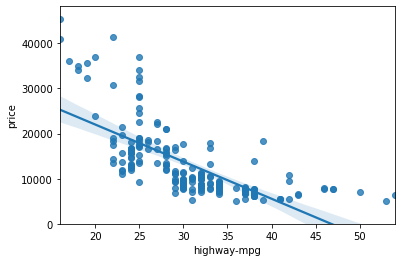We can see from this plot that price is negatively correlated to highway-mpg, since the regression slope is negative. One thing to keep in mind when looking at a regression plot is to pay attention to how scattered the data points are around the regression line. This will give you a good indication of the variance of the data, and whether a linear model would be the best fit or not. If the data is too far off from the line, this linear model might not be the best model for this data.

Residual Plot

A good way to visualize the variance of the data is to use a residual plot.

What is a residual?

The difference between the observed value (y) and the predicted value (Yhat) is called the residual (e). When we look at a regression plot, the residual is the distance from the data point to the fitted regression line.

So what is a residual plot?

A residual plot is a graph that shows the residuals on the vertical y-axis and the independent variable on the horizontal x-axis.

What do we pay attention to when looking at a residual plot?

We look at the spread of the residuals:

• If the points in a residual plot are randomly spread out around the x-axis, then a linear model is appropriate for the data. Why is that? Randomly spread out residuals means that the variance is constant, and thus the linear model is a good fit for this data.
width = 12
height = 10
plt.figure(figsize=(width, height))
sns.residplot(df['highway-mpg'], df['price'])
plt.show()
/opt/conda/lib/python3.8/site-packages/seaborn/_decorators.py:36: FutureWarning: Pass the following variables as keyword args: x, y. From version 0.12, the only valid positional argument will be `data`, and passing other arguments without an explicit keyword will result in an error or misinterpretation. warnings.warn(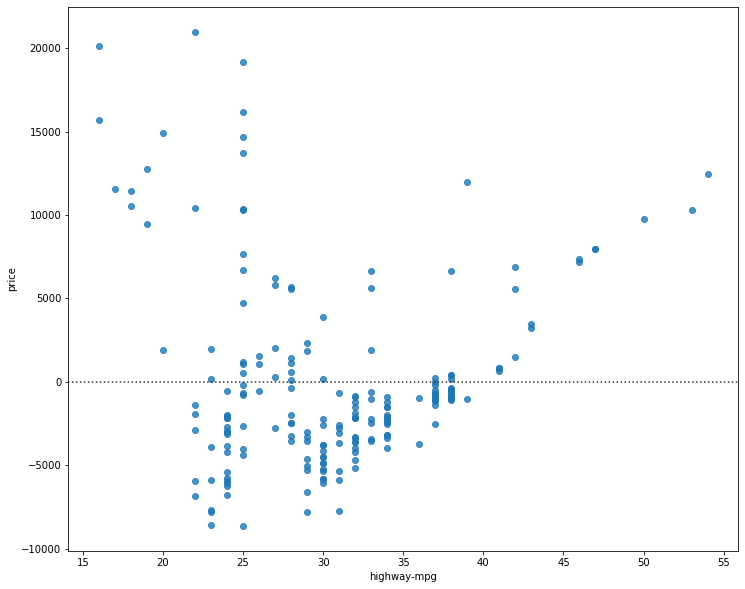What is this plot telling us?

We can see from this residual plot that the residuals are not randomly spread around the x-axis, which leads us to believe that maybe a non-linear model is more appropriate for this data.

Multiple Linear Regression

How do we visualize a model for Multiple Linear Regression? This gets a bit more complicated because you can't visualize it with regression or residual plot.

One way to look at the fit of the model is by looking at the distribution plot: We can look at the distribution of the fitted values that result from the model and compare it to the distribution of the actual values.

First lets make a prediction

Y_hat = lm.predict(df[['highway-mpg']])
print('Simple Linear Regressionn')

# plot
plt.figure(figsize=(width, height))

ax1 = sns.distplot(df['price'], hist=False, color="r")
sns.distplot(Y_hat, hist=False, color="b", ax=ax1)

plt.legend(["Actual Value","Fitted Values"])
plt.title('Actual vs Fitted Values for Price')
plt.xlabel('Price (in dollars)')
plt.ylabel('Proportion of Cars')

plt.show()
plt.close()
Simple Linear Regressionn
/opt/conda/lib/python3.8/site-packages/seaborn/distributions.py:2551: FutureWarning: `distplot` is a deprecated function and will be removed in a future version. Please adapt your code to use either `displot` (a figure-level function with similar flexibility) or `kdeplot` (an axes-level function for kernel density plots). warnings.warn(msg, FutureWarning) /opt/conda/lib/python3.8/site-packages/seaborn/distributions.py:2551: FutureWarning: `distplot` is a deprecated function and will be removed in a future version. Please adapt your code to use either `displot` (a figure-level function with similar flexibility) or `kdeplot` (an axes-level function for kernel density plots). warnings.warn(msg, FutureWarning)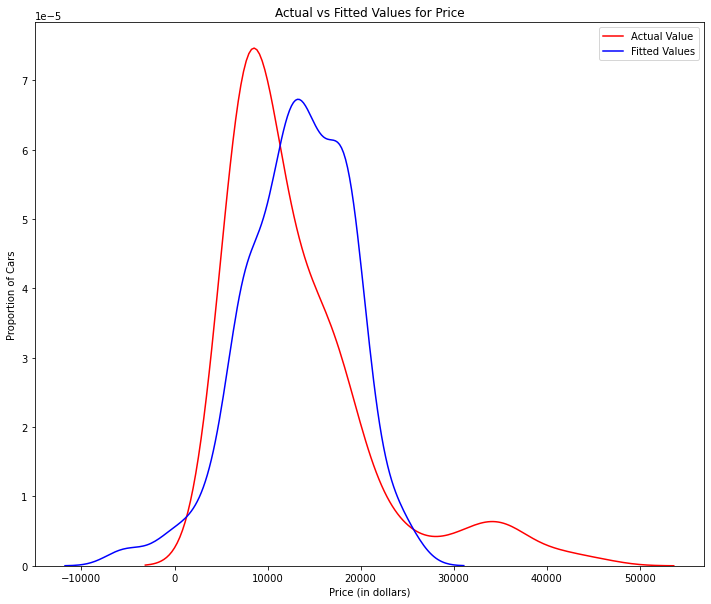Y_hat = lm3.predict(df[['horsepower', 'curb-weight', 'engine-size', 'highway-mpg']])
print("Multiple Linear Regression")
# plot
plt.figure(figsize=(width, height))

ax1 = sns.distplot(df['price'], hist=False, color="r")
sns.distplot(Y_hat, hist=False, color="b", ax=ax1)

plt.legend(["Actual Value","Fitted Values"])
plt.title('Actual vs Fitted Values for Price')
plt.xlabel('Price (in dollars)')
plt.ylabel('Proportion of Cars')

plt.show()
plt.close()
Multiple Linear Regression
/opt/conda/lib/python3.8/site-packages/seaborn/distributions.py:2551: FutureWarning: `distplot` is a deprecated function and will be removed in a future version. Please adapt your code to use either `displot` (a figure-level function with similar flexibility) or `kdeplot` (an axes-level function for kernel density plots). warnings.warn(msg, FutureWarning) /opt/conda/lib/python3.8/site-packages/seaborn/distributions.py:2551: FutureWarning: `distplot` is a deprecated function and will be removed in a future version. Please adapt your code to use either `displot` (a figure-level function with similar flexibility) or `kdeplot` (an axes-level function for kernel density plots). warnings.warn(msg, FutureWarning)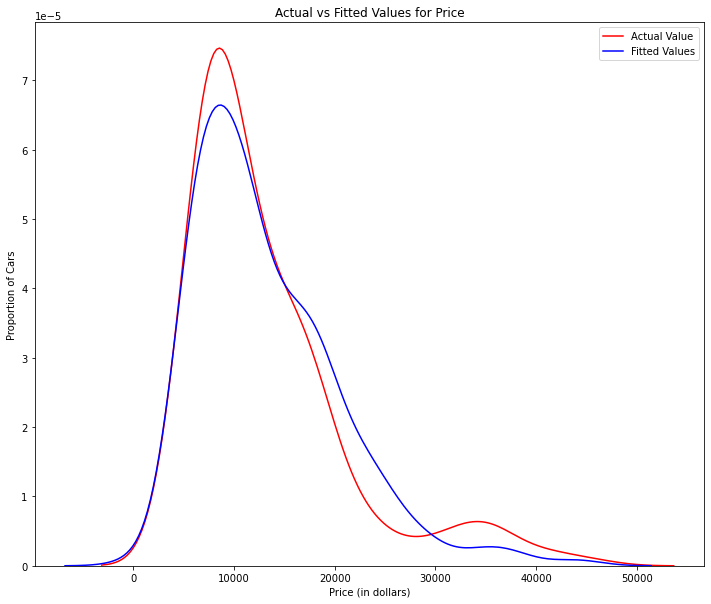We can see that the fitted values are reasonably close to the actual values, since the two distributions overlap a bit. However, there is definitely some room for improvement.

3: Polynomial Regression and Pipeline

Polynomial regression is a particular case of the general linear regression model or multiple linear regression models.

We get non-linear relationships by squaring or setting higher-order terms of the predictor variables.

There are different orders of polynomial regression:

\$\$ Yhat = a + b_1 X +b_2 X^2 \$\$
Cubic - 3rd order
\$\$ Yhat = a + b_1 X +b_2 X^2 +b_3 X^3\\\\ \$\$
Higher order:
\$\$ Y = a + b_1 X +b_2 X^2 +b_3 X^3 ....\\\\ \$\$

We saw earlier that a linear model did not provide the best fit while using highway-mpg as the predictor variable. Let's see if we can try fitting a polynomial model to the data instead.

We will use the following function to plot the data:

def Polly_Plot(model, independent_variable, dependent_variabble, Name):
x_new = np.linspace(15, 55, 100)
y_new = model(x_new)

plt.plot(independent_variable, dependent_variabble, '.', x_new, y_new, '-')
plt.title('Polynomial Fit with Matplotlib for Price ~ Length')
ax = plt.gca()
ax.set_facecolor((0.898, 0.898, 0.898))
fig = plt.gcf()
plt.xlabel(Name)
plt.ylabel('Price of Cars')

plt.show()
plt.close()
# Lets get the variables
x = df['highway-mpg']
y = df['price']

# Here we use a polynomial of the 3rd order (cubic)
f = np.polyfit(x, y, 3)
p = np.poly1d(f)
print(p)
3 2 -1.557 x + 204.8 x - 8965 x + 1.379e+05

Let's plot the function

Polly_Plot(p, x, y, 'highway-mpg')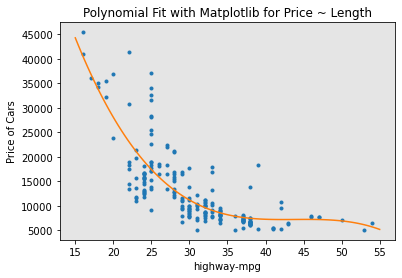We can already see from plotting that this polynomial model performs better than the linear model. This is because the generated polynomial function hits more of the data points.

Create 11 order polynomial model with the variables x and y from above

f1 = np.polyfit(x, y, 11)
p1 = np.poly1d(f1)
print(p1)
Polly_Plot(p1,x,y, 'Highway MPG')
11 10 9 8 7 -1.243e-08 x + 4.722e-06 x - 0.0008028 x + 0.08056 x - 5.297 x 6 5 4 3 2 + 239.5 x - 7588 x + 1.684e+05 x - 2.565e+06 x + 2.551e+07 x - 1.491e+08 x + 3.879e+08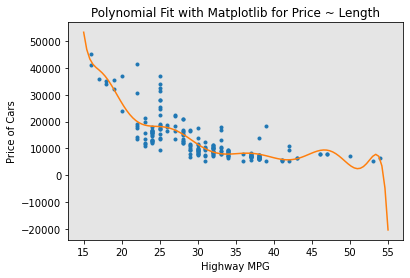The analytical expression for Multivariate Polynomial function gets complicated. For example, the expression for a second-order (degree=2)polynomial with two variables is given by:

\[ Yhat = a + b_1 X_1 +b_2 X_2 +b_3 X_1 X_2+b_4 X_1^2+b_5 X_2^2 \]

We can perform a polynomial transform on multiple features. First, we import the module:

from sklearn.preprocessing import PolynomialFeatures

We create a PolynomialFeatures object of degree 2:

pr=PolynomialFeatures(degree=2)
pr
PolynomialFeatures()
Z_pr=pr.fit_transform(Z)

The original data is of 201 samples and 4 features

Z.shape
(201, 4)

after the transformation, there 201 samples and 15 features

Z_pr.shape
(201, 15)

Pipeline

Data Pipelines simplify the steps of processing the data. We use the module Pipeline to create a pipeline. We also use StandardScaler - to Normalize the data as a step in our pipeline .

from sklearn.pipeline import Pipeline
from sklearn.preprocessing import StandardScaler

We create the pipeline, by creating a list of tuples including the name of the model or estimator and its corresponding constructor.

Input=[('scale',StandardScaler()), ('polynomial', PolynomialFeatures(include_bias=False)), ('model',LinearRegression())]

we input the list as an argument to the pipeline constructor

pipe=Pipeline(Input)
pipe
Pipeline(steps=[('scale', StandardScaler()),
('polynomial', PolynomialFeatures(include_bias=False)),
('model', LinearRegression())])

We can normalize the data, perform a transform and fit the model simultaneously.

pipe.fit(Z,y)
Pipeline(steps=[('scale', StandardScaler()),
('polynomial', PolynomialFeatures(include_bias=False)),
('model', LinearRegression())])

Similarly, we can normalize the data, perform a transform and produce a prediction simultaneously

ypipe=pipe.predict(Z)
ypipe[0:4]
array([13102.93329646, 13102.93329646, 18226.43450275, 10391.09183955])

4: Measures for In-Sample Evaluation

When evaluating our models, not only do we want to visualize the results, but we also want a quantitative measure to determine how accurate the model is.

Two very important measures that are often used in Statistics to determine the accuracy of a model are:

• R^2 / R-squared
• Mean Squared Error (MSE)

R-squared R squared, also known as the coefficient of determination, is a measure to indicate how close the data is to the fitted regression line.

The value of the R-squared is the percentage of variation of the response variable (y) that is explained by a linear model.

Mean Squared Error (MSE)

The Mean Squared Error measures the average of the squares of errors, that is, the difference between actual value (y) and the estimated value (ŷ).

Let's calculate the R^2

#highway_mpg_fit
X = df[['highway-mpg']]
Y = df['price']
lm.fit(X, Y)
# Find the R^2
print('The R-square is: ', lm.score(X, Y))
The R-square is: 0.4965911884339176

We can say that ~ 49.659% of the variation of the price is explained by this simple linear model horsepower_fit.

To calculate the MSE

lets import the function mean_squared_error from the module metrics

from sklearn.metrics import mean_squared_error

we compare the predicted results with the actual results

Yhat=lm.predict(X)
mse = mean_squared_error(df['price'], Yhat)
print('The mean square error of price and predicted value is: ', mse)
The mean square error of price and predicted value is: 31635042.944639888
Model 2: Multiple Linear Regression

Let's calculate the R^2

# fit the model
lm.fit(Z, df['price'])
# Find the R^2
print('The R-square is: ', lm.score(Z, df['price']))
The R-square is: 0.8093732522175299

We can say that ~ 80.896 % of the variation of price is explained by this multiple linear regression "multi_fit".

Let's calculate the MSE

we produce a prediction

Y_predict_multifit = lm.predict(Z)

we compare the predicted results with the actual results

print('The mean square error of price and predicted value using multifit is: ', \
mean_squared_error(df['price'], Y_predict_multifit))
The mean square error of price and predicted value using multifit is: 11979300.34981888

Model 3: Polynomial Fit

To calculate the R^2, let’s import the function r2_score from the module metrics as we are using a different function

from sklearn.metrics import r2_score

We apply the function to get the value of r^2

r_squared = r2_score(y, p(x)) # degree 3 polynomial
print('The R-square value is: ', r_squared)
The R-square value is: 0.674194666390652

We can say that ~ 67.419 % of the variation of price is explained by this polynomial fit

We can also calculate the MSE:

mean_squared_error(df['price'], p(x)) # degree 3 polynomial
20474146.426361218
r_squared = r2_score(y, p1(x)) #  degree 11 polynomial
print('The R-square value is: ', r_squared)
The R-square value is: 0.702376909243598
mean_squared_error(df['price'], p1(x)) # degree 11 polynomial
18703127.63915394

5: Prediction and Decision Making

Prediction

In the previous section, we trained the model using the method fit. Now we will use the method predict to produce a prediction. we will use pyplot for plotting, also be using some functions from numpy.

Create a new input

new_input=np.arange(1, 101, 1).reshape(-1, 1) # 100 sample inputs

Fit the model

lm.fit(X, Y)
lm
LinearRegression()

Produce a prediction

yhat=lm.predict(new_input)
yhat[0:5]
array([37601.57247984, 36779.83910151, 35958.10572319, 35136.37234487,
34314.63896655])

we can plot the data

plt.plot(new_input, yhat)
plt.show()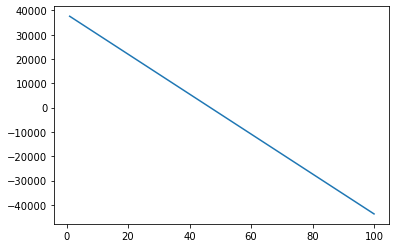Decision Making: Determining a Good Model Fit

Now that we have visualized the different models, and generated the R-squared and MSE values for the fits, how do we determine a good model fit?

What is a good R-squared value? When comparing models, the model with the higher R-squared value is a better fit for the data.

What is a good MSE? When comparing models, the model with the smallest MSE value is a better fit for the data.

Let's take a look at the values for the different models.

Simple Linear Regression: Using Highway-mpg as a Predictor Variable of Price.

• R-squared: 0.49659118843391759
• MSE: 3.16 x10^7

Multiple Linear Regression: Using Horsepower, Curb-weight, Engine-size, and Highway-mpg as Predictor Variables of Price.

• R-squared: 0.80896354913783497
• MSE: 1.2 x10^7

Polynomial Fit: Using Highway-mpg as a Predictor Variable of Price.

• R-squared: 0.6741946663906514
• MSE: 2.05 x 10^7

Simple Linear Regression model (SLR) vs Multiple Linear Regression model (MLR) Usually, the more variables you have, the better your model is at predicting, but this is not always true. Sometimes you may not have enough data, you may run into numerical problems, or many of the variables may not be useful and or even act as noise. As a result, you should always check the MSE and R^2.

So to be able to compare the results of the MLR vs SLR models, we look at a combination of both the R-squared and MSE to make the best conclusion about the fit of the model.

• MSE: The MSE of SLR is 3.16x10^7 while MLR has an MSE of 1.2 x10^7. The MSE of MLR is much smaller.
• R-squared: In this case, we can also see that there is a big difference between the R-squared of the SLR and the R-squared of the MLR. The R-squared for the SLR (0.497) is very small compared to the R-squared for the MLR (0.809).

This R-squared in combination with the MSE show that MLR seems like the better model fit in this case, compared to SLR.

Simple Linear Model (SLR) vs Polynomial Fit

• MSE: We can see that Polynomial Fit brought down the MSE, since this MSE is smaller than the one from the SLR.
• R-squared: The R-squared for the Polyfit is larger than the R-squared for the SLR, so the Polynomial Fit also brought up the R-squared quite a bit.

Since the Polynomial Fit resulted in a lower MSE and a higher R-squared, we can conclude that this was a better fit model than the simple linear regression for predicting Price with Highway-mpg as a predictor variable.

Multiple Linear Regression (MLR) vs Polynomial Fit

• MSE: The MSE for the MLR is smaller than the MSE for the Polynomial Fit.
• R-squared: The R-squared for the MLR is also much larger than for the Polynomial Fit.

Model Evaluation and Refinement

In the previous section, we have evalulated the model using in-sample evaluation. In-sample evaluation tells us how well our model fits the data already given to train it. It does not give us an estimate of how well the train model can predict new data. The solution is to split our data up, use the in-sample data or training data to train the model. The rest of the data, called Test Data, is used as out-of-sample data. This data is then used to approximate, how the model performs in the real world. Separating data into training and testing sets is an important part of model evaluation. We use the test data to get an idea how our model will perform in the real world.

Functions for Plotting

def DistributionPlot(RedFunction, BlueFunction, RedName, BlueName, Title): # red function : actual, Blue Function : predicted
width = 12
height = 10
plt.figure(figsize=(width, height))

ax1 = sns.distplot(RedFunction, hist=False, color="r", label=RedName)
ax2 = sns.distplot(BlueFunction, hist=False, color="b", label=BlueName, ax=ax1)

plt.title(Title)
plt.xlabel('Price (in dollars)')
plt.ylabel('Proportion of Cars')
plt.legend([RedName,BlueName])

plt.show()
plt.close()
def PollyPlot(xtrain, xtest, y_train, y_test, lr,poly_transform):
width = 12
height = 10
plt.figure(figsize=(width, height))

#training data
#testing data
# lr:  linear regression object
#poly_transform:  polynomial transformation object

xmax=max([xtrain.values.max(), xtest.values.max()])

xmin=min([xtrain.values.min(), xtest.values.min()])

x=np.arange(xmin, xmax, 0.1)

plt.plot(xtrain, y_train, 'or', label='Training Data')
plt.plot(xtest, y_test, 'og', label='Test Data')
plt.plot(x, lr.predict(poly_transform.fit_transform(x.reshape(-1, 1))), label='Predicted Function')
plt.ylim([-10000, 60000])
plt.ylabel('Price')
plt.legend()

Part 1: Training and Testing

An important step in testing your model is to split your data into training and testing data.

# We will place the target data price in a separate dataframe y:
y_data = df['price']

#drop price data in x data
x_data = df.drop('price',axis=1)

Now we randomly split our data into training and testing data using the function train_test_split.

from sklearn.model_selection import train_test_split

x_train, x_test, y_train, y_test = train_test_split(x_data, y_data, test_size=0.10, random_state=1)

print("number of test samples :", x_test.shape)
print("number of training samples:",x_train.shape)
number of test samples : 21 number of training samples: 180

The test_size parameter sets the proportion of data that is split into the testing set. In the above, the testing set is set to 10% of the total dataset.

Let's Calculate the R^2 on the test data (x_train, x_test, y_train, y_test):

# let's import LinearRegression from the module linear_model.
from sklearn.linear_model import LinearRegression

#We create a Linear Regression object:
lre=LinearRegression()

#we fit the model using the feature horsepower
lre.fit(x_train[['horsepower']], y_train)

# Let's Calculate the R^2 on the test data:
test =  lre.score(x_test[['horsepower']], y_test)
print('the R^2 on the Test data:', test)

# Let's Calculate the R^2 on the Train data:
train = lre.score(x_train[['horsepower']], y_train)
print('the R^2 on the Train data:', train)
the R^2 on the Test data: 0.3635480624962414 the R^2 on the Train data: 0.662028747521533

we can see the R^2 is much smaller using the test data. This is because almost 90% of the data is used to train the model becuase of which the model is able of explain 66% of the seen data(i.e. 90% of the total data) and only 10% of the data to test it because of which it can explain only 36% of that unseen 10% data. In short, the model has good accuracy but very low precision.

Now, let's split up the data set such that 40% of the data samples will be utilized for testing, set the parameter "random_state" equal to zero. The output of the function should be the following: "x_train_1" , "x_test_1", "y_train_1" and "y_test_1"

x_train1,x_test1, y_train1,  y_test1 = train_test_split(x_data, y_data,test_size=0.4, random_state=0 )
print("number of test samples :", x_test1.shape)
print("number of training samples:",x_train1.shape)

# training model and calculating R^2
lre1=LinearRegression()
lre1.fit(x_train1[['horsepower']], y_train1)
# Let's Calculate the R^2 on the test data:
test1 =  lre1.score(x_test1[['horsepower']], y_test1)
print('the R^2 on the Test data:', test1)

# Let's Calculate the R^2 on the Train data:
train1 = lre1.score(x_train1[['horsepower']], y_train1)
print('the R^2 on the Train data:', train1)
number of test samples : 81 number of training samples: 120 the R^2 on the Test data: 0.7139737368233017 the R^2 on the Train data: 0.5754853866574969

We can see a big jump precision to 71% from 36% and slight drop in accuracy from 66% to 57%. But this model can predict real world data more precisely.

Cross-validation Score

Sometimes you do not have sufficient testing data; as a result, you may want to perform Cross-validation. Let's go over several methods that you can use for Cross-validation.

# Lets import model_selection from the module cross_val_score.
from sklearn.model_selection import cross_val_score

# We input the object, the feature in this case ' horsepower', the target data (y_data).
# The parameter 'cv' determines the number of folds; in this case 4.
Rcross = cross_val_score(lre, x_data[['horsepower']], y_data, cv=4)

# The default scoring is R^2; each element in the array has the average R^2 value in the fold:
Rcross
array([0.77465419, 0.51718424, 0.74814454, 0.04825398])

We can calculate the average and standard deviation of our estimate:

print("The mean of the folds are", Rcross.mean(), "and the standard deviation is" , Rcross.std())
The mean of the folds are 0.5220592359225417 and the standard deviation is 0.2913048066611841

You can also use the function cross_val_predict to predict the output. The function splits up the data into the specified number of folds, using one fold for testing and the other folds are used for training. First import the function:

from sklearn.model_selection import cross_val_predict

We input the object, the feature in this case horsepower , the target data y_data. The parameter cv determines the number of folds; in this case 4. We can produce an output that was obtained for each element when it was in the test set.:

yhat = cross_val_predict(lre,x_data[['horsepower']], y_data,cv=4)
yhat[0:5] # yhat has total 201 values
array([14142.23793549, 14142.23793549, 20815.3029844 , 12745.549902  ,
14762.9881726 ])

Part 2: Overfitting, Underfitting and Model Selection

It turns out that the test data sometimes referred to as the out of sample data is a much better measure of how well your model performs in the real world. One reason for this is overfitting; let's go over some examples. It turns out these differences are more apparent in Multiple Linear Regression and Polynomial Regression so we will explore overfitting in that context.

Let's create Multiple linear regression objects and train the model using 'horsepower', 'curb-weight', 'engine-size' and 'highway-mpg' as features.

lr = LinearRegression()
lr.fit(x_train[['horsepower', 'curb-weight', 'engine-size', 'highway-mpg']], y_train) # 10% test data
LinearRegression()

Prediction using training data:

yhat_train = lr.predict(x_train[['horsepower', 'curb-weight', 'engine-size', 'highway-mpg']])
yhat_train[0:5]
array([ 7426.34910902, 28324.42490838, 14212.74872339,  4052.80810192,
34499.8541269 ])

Prediction using test data:

yhat_test = lr.predict(x_test[['horsepower', 'curb-weight', 'engine-size', 'highway-mpg']])
yhat_test[0:5]
array([11349.68099115,  5884.25292475, 11208.31007475,  6641.03017109,
15565.98722248])

Let's perform some model evaluation using our training and testing data separately.

Title = 'Distribution  Plot of  Predicted Value Using Training Data vs Training Data Distribution'
DistributionPlot(y_train, yhat_train, "Actual Values (Train)", "Predicted Values (Train)", Title)
/opt/conda/lib/python3.8/site-packages/seaborn/distributions.py:2551: FutureWarning: `distplot` is a deprecated function and will be removed in a future version. Please adapt your code to use either `displot` (a figure-level function with similar flexibility) or `kdeplot` (an axes-level function for kernel density plots). warnings.warn(msg, FutureWarning) /opt/conda/lib/python3.8/site-packages/seaborn/distributions.py:2551: FutureWarning: `distplot` is a deprecated function and will be removed in a future version. Please adapt your code to use either `displot` (a figure-level function with similar flexibility) or `kdeplot` (an axes-level function for kernel density plots). warnings.warn(msg, FutureWarning)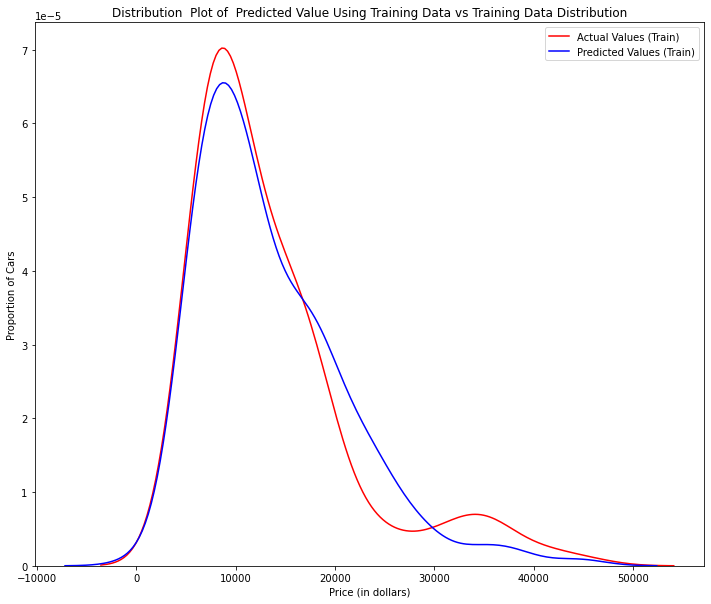Figure 1: Plot of predicted values using the training data compared to the training data.

So far the model seems to be doing well in learning from the training dataset. But what happens when the model encounters new data from the testing dataset? When the model generates new values from the test data, we see the distribution of the predicted values is much different from the actual target values.

Title='Distribution  Plot of  Predicted Value Using Test Data vs Data Distribution of Test Data'
DistributionPlot(y_test,yhat_test,"Actual Values (Test)","Predicted Values (Test)",Title)
/opt/conda/lib/python3.8/site-packages/seaborn/distributions.py:2551: FutureWarning: `distplot` is a deprecated function and will be removed in a future version. Please adapt your code to use either `displot` (a figure-level function with similar flexibility) or `kdeplot` (an axes-level function for kernel density plots). warnings.warn(msg, FutureWarning) /opt/conda/lib/python3.8/site-packages/seaborn/distributions.py:2551: FutureWarning: `distplot` is a deprecated function and will be removed in a future version. Please adapt your code to use either `displot` (a figure-level function with similar flexibility) or `kdeplot` (an axes-level function for kernel density plots). warnings.warn(msg, FutureWarning)